Next: 5. Photoresist Exposure/Bleaching Simulation Up: 4.4 Numerical Implementation Previous: 4.4.1 Alias Free'' Forward

## 4.4.2 Numerical Backward Transform

In (4.51) and (4.55) we showed that the source point contributions to the aerial image are obtained by Fourier transforms like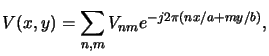(4.97)

whereby V(x, y) vicariously stands for the scalar or vector amplitude Upqi(x, y) and Epqi(x, y), respectively. The spectrum Vnm of the source point contribution is given either in (4.52) or (4.57) depending on the chosen theory. In both cases it is determined by the following three quantities: source amplitude Apq, Fourier coefficients Tnm of the mask transfer function, and discrete pupil function P(n, m) or P(n, m : p, q). For each of them formulae are provided in the preceding sections. Hence, the spectrum can be calculated and the field is obtained by evaluating the transform in (4.107). This last step is accomplished as follows.

The finite extent of the pupil function in (4.53) or (4.58) shows that the image spectrum is always a low-pass function, i.e.,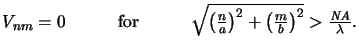(4.98)

Hence, the fundamental results of the sampling theory [129,130] can be applied, which state that any band-limited, finite-energy function f (x) can be reconstructed from discrete equally-spaced samples f (n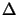x), if the spacing between the samplesx meets the Nyquist criterionx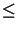1/2W, whereby W is the highest frequency occurring in f (x). Self-evidently the sampling theorem is also valid for functions defined in a higher dimensional space. As can be seen from (4.108) the aerial image spectrum Vnm is circularly band-limited with cut-off frequency NA/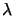. Consequently, it suffices to evaluate the field V(x, y) at discrete points only without any loss of information. We choose the spacingsx andy to meet the Nyquist criterion and require that the numbers of samples within the mask periods Nx and Ny equal a power of two,q i.e.,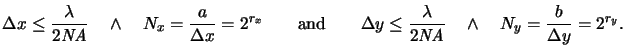(4.99)

Thus the powers rx = ldNx and ry = ldNy are chosen as the smallest integers meeting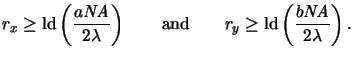(4.100)

The field disturbance on the equally spaced ortho-product grid is given now by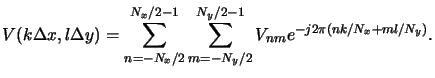(4.101)

This formula can efficiently be evaluated with a radix-2 FFT algorithm [131, ch. 9], [132, ch. 12], [133, ch. 11]. We have chosen a public-domain software package that employs heavily inlined FFT routines and precalculated weight tables to achieve maximal speed.r The length of the weight tables is 4096 and the unroll value is 128. Above the unroll value conventional recursive routines are used. To check the correctness of the algorithm we compared the results with the well-established packet FFTPACK  from the NETLIB.s

Information on the computational performance of the aerial image tool, e.g., run-time and memory consumption, is given in connection with simulation examples in Chapter 8.1.

#### Footnotes

... two,q
The second requirement is not obligatory, but the resulting formulae can then be evaluated with the most efficient radix-2 FFT algorithm.
... speed.r
This package was written by Richard Krukar and is available at http://hpux.u-aizu.ac.jp/hppd/hpux/Maths/Misc/ffts_in_C-1.0/readme.html.
... NETLIB.s
The package FFTPACK was written by Paul N. Swarztrauber and is widely available, e.g., from the original NETLIB location at http://www.netlib.org/fftpack/index.html.Next: 5. Photoresist Exposure/Bleaching Simulation Up: 4.4 Numerical Implementation Previous: 4.4.1 Alias Free'' Forward
Heinrich Kirchauer, Institute for Microelectronics, TU Vienna
1998-04-17